# Soil water flow and Darcys Law Laminar flow

• Slides: 22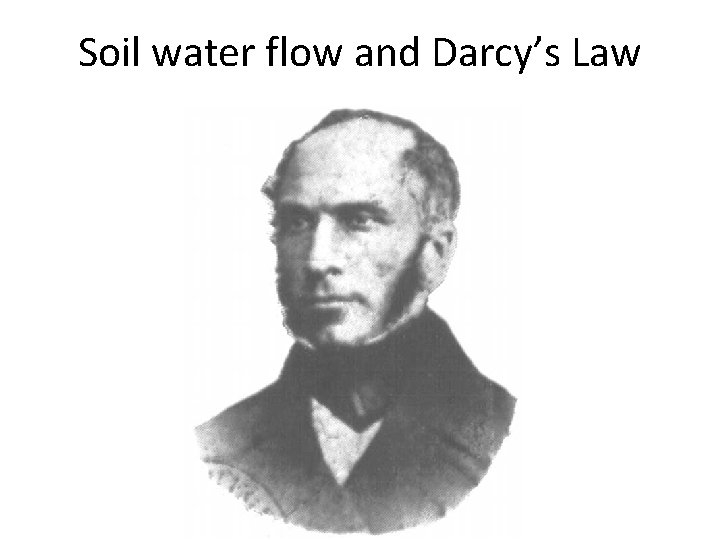Soil water flow and Darcy’s Law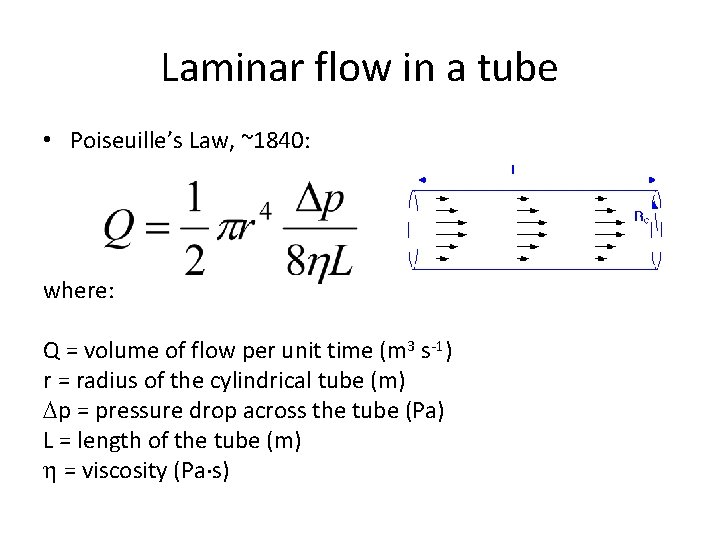Laminar flow in a tube • Poiseuille’s Law, ~1840: where: Q = volume of flow per unit time (m 3 s-1) r = radius of the cylindrical tube (m) p = pressure drop across the tube (Pa) L = length of the tube (m) = viscosity (Pa s)Macroscopic flow-velocity vector • the overall average of the microscopic velocities over the total volume considered • the volume considered must be large relative to the scale of heterogeneity • the ratio of the path length through the soil pores to the net travel distance is the tortuosity• insert fig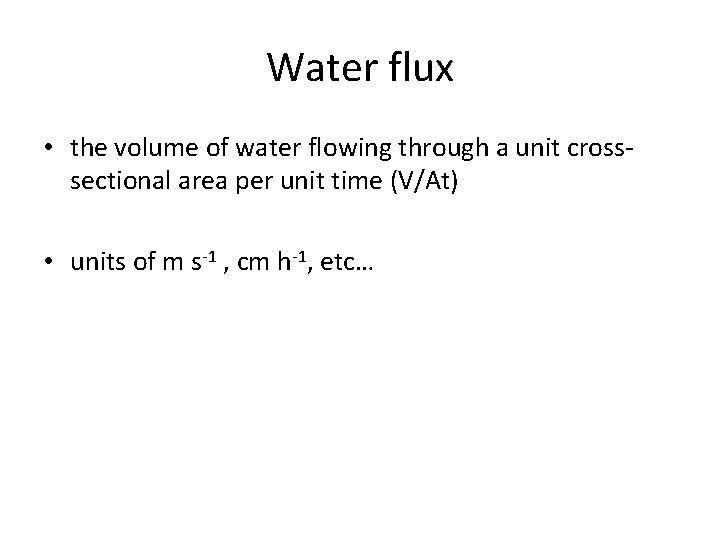Water flux • the volume of water flowing through a unit crosssectional area per unit time (V/At) • units of m s-1 , cm h-1, etc…Hydraulic gradient • the drop in water potential per unit distance in the direction of flow ( H/L) • a driving force for water flow • units depend on how water potential is specified a) commonly water potential is in units of “head” (e. g. cm of water) and the hydraulic gradient is unitless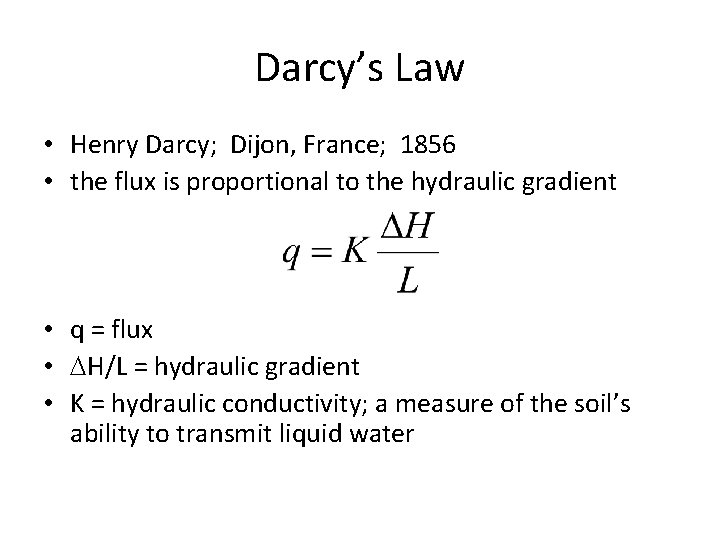Darcy’s Law • Henry Darcy; Dijon, France; 1856 • the flux is proportional to the hydraulic gradient • q = flux • H/L = hydraulic gradient • K = hydraulic conductivity; a measure of the soil’s ability to transmit liquid water• Hpi = 100 cm; Hpo = 60 cm; L = 50 cm; K = 10 cm h-1 • q=?• H 1= 10 cm; L = 50 cm; K = 10 cm h-1 • q=?Reading assignment • p. 137 -146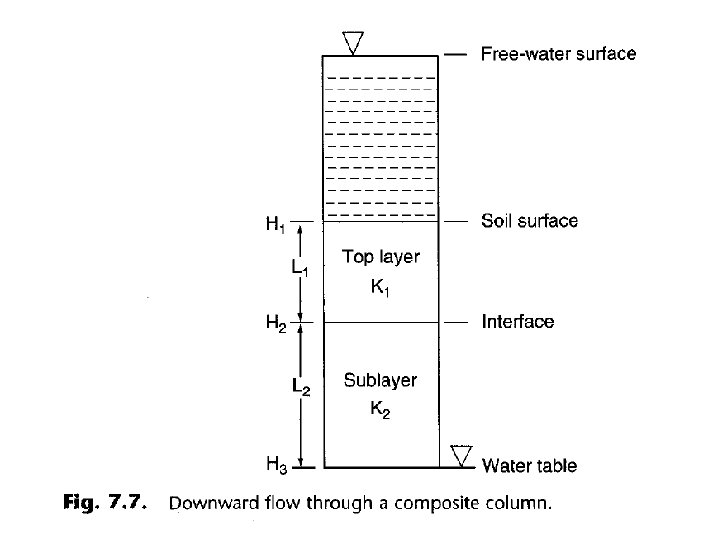• insert Fig. 7. 7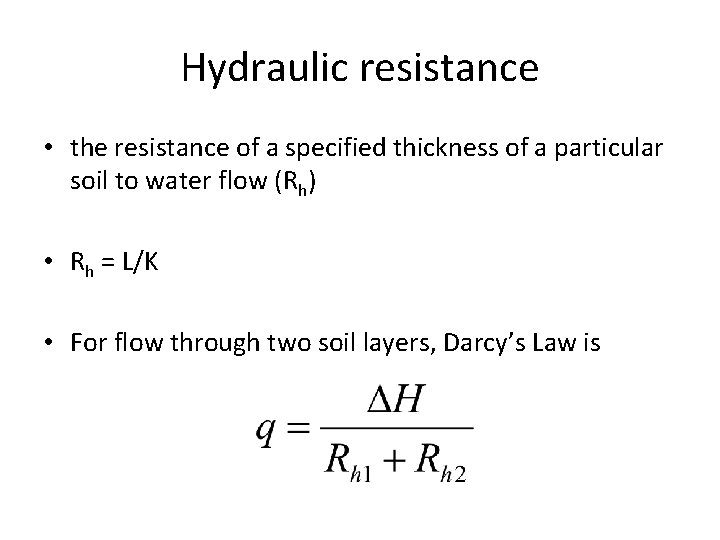Hydraulic resistance • the resistance of a specified thickness of a particular soil to water flow (Rh) • Rh = L/K • For flow through two soil layers, Darcy’s Law is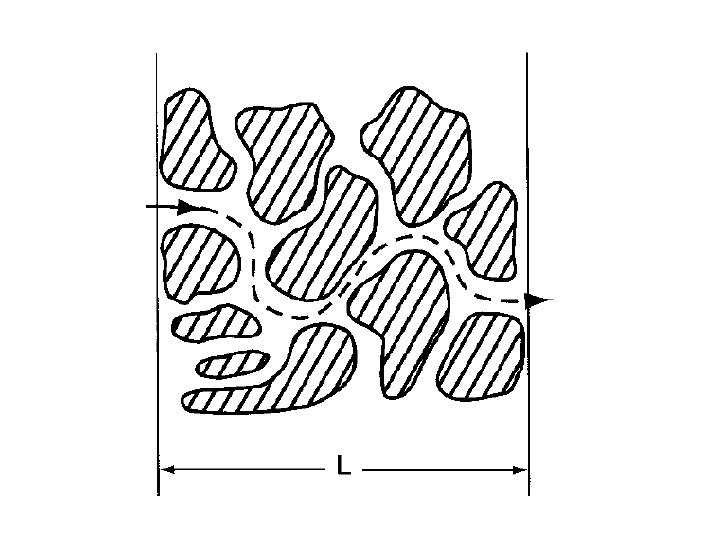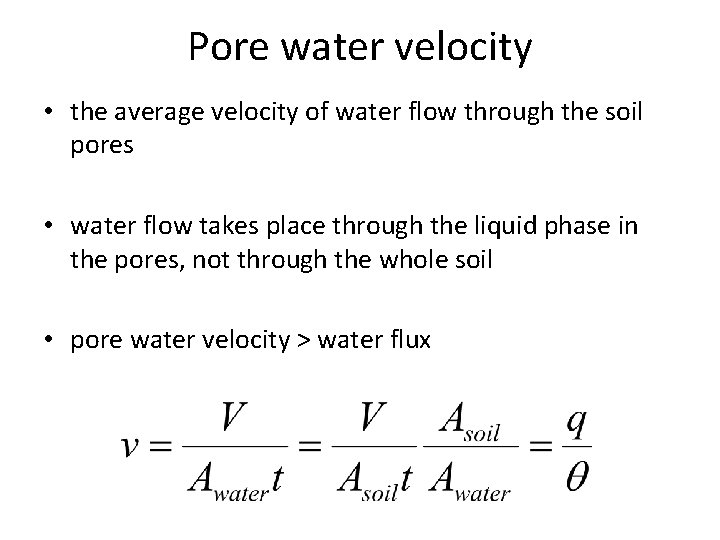Pore water velocity • the average velocity of water flow through the soil pores • water flow takes place through the liquid phase in the pores, not through the whole soil • pore water velocity > water flux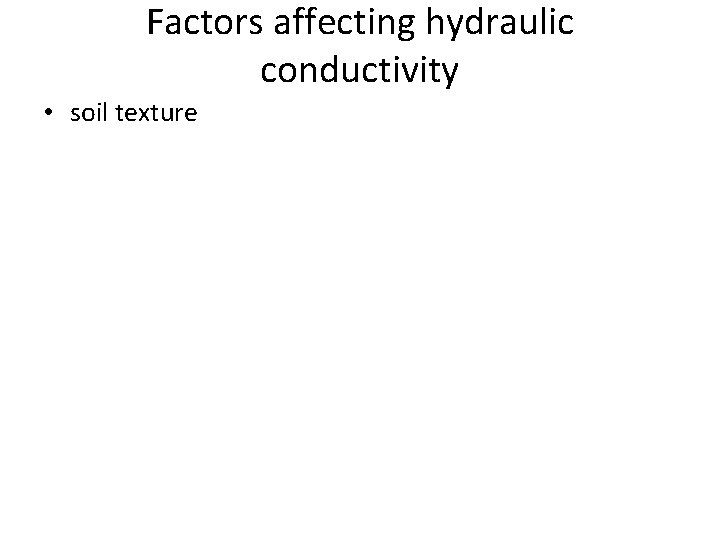Factors affecting hydraulic conductivity • soil texture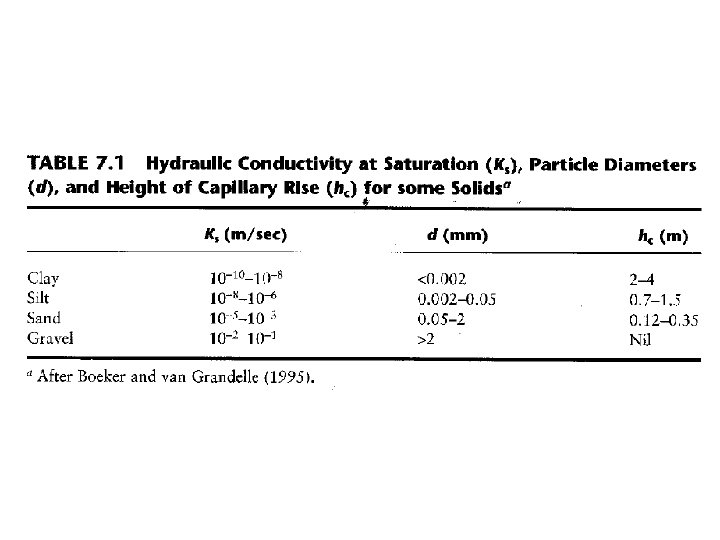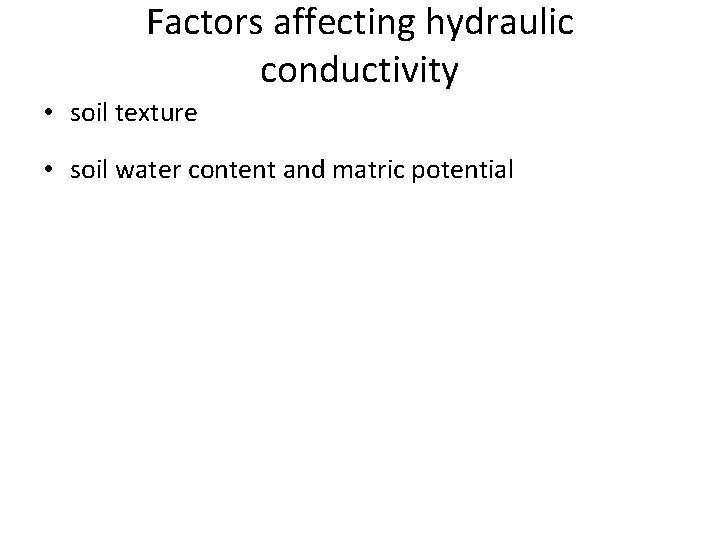Factors affecting hydraulic conductivity • soil texture • soil water content and matric potentialFactors affecting hydraulic conductivity • soil texture • soil water content and matric potential • soil structure • electrical conductivity of the soil solution • species of cations present • entrapped air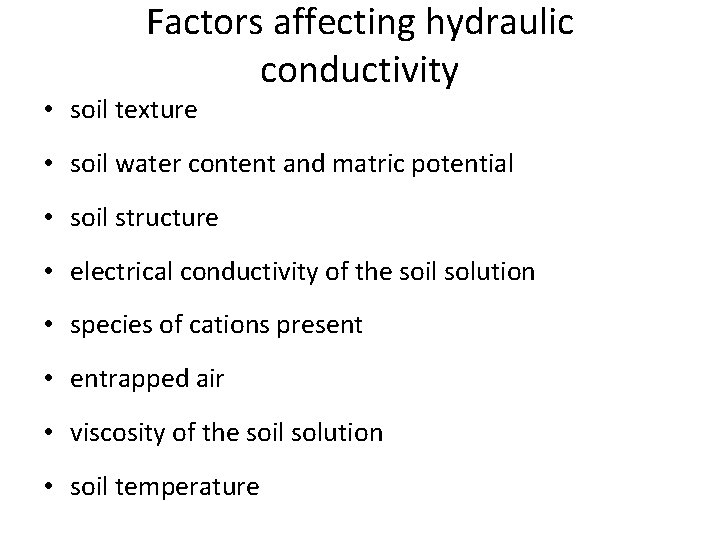Factors affecting hydraulic conductivity • soil texture • soil water content and matric potential • soil structure • electrical conductivity of the soil solution • species of cations present • entrapped air • viscosity of the soil solution • soil temperatureReading assignment • Infiltration, p. 259 -265